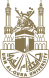# جامعة أم القرى# Course Description- Theoretical Methods 1

- 2018/01/27

Course Description

Infinite Series, Power Series

• The Geometric Series
• Definitions and Notation
• Convergent and Divergent Series
• Testing Series for Convergence; the Preliminary Test
• Convergence Tests for Series of Positive Terms: Absolute Convergence
• Alternating Series
• Power Series; Interval of Convergence
• Expanding Functions in Power Series
• Techniques for Obtaining Power Series Expansions
• Some Uses of Series

Partial Differentation

• Introduction and Notation
• Power Series in Two Variables
• Total Differentials
• Approximations using Differentials
• Chain Rule or Differentiating a Function of a Function
• Implicit Differentiation
• Maximum and Minimum Problems with Constraints; Lagrange Multipliers
• Change of Variables
• Differentiation of Integrals; Leibniz' Rule

Vector Analysis

• Introduction
• Application of Vector Multiplication
• Triple Products
• Differentiation of Vectors
• Fields
• ∇  Some Other Expressions involving
• Line Integrals
• Green's Theorem in the plane
• The Divergence and the Divergence Theorem
• The Curl and Stokes' Theorem

Fourier Series and Transforms

• Introduction
• Simple Harmonic Motion and Wave Motion; Periodic Functions
• Applications of Fourier Series
• A verage Value of a Function
• Fourier Coefficients
• Complex Form of Fourier Series
• Other Intervals
• Even and Odd Functions
• An Application to Sound
• Parseval's Theorem
• Fourier Transforms

Ordinary Differential Equations and The Laplace Transforms

• Introduction
• Separable Equations
• Linear First-Order Equations
• Other Methods for First-Order Equations
• Second-Order Linear Equations with Constant Coefficients and Zero Right-Hand Side
• Second-Order Linear Equations with Constant Coefficients and Right-Hand Side
• The Laplace Transform
• Solution of Differential Equations by Laplace Transforms
• Convolution
• The Dirac-Delta Function
• A Brief Introduction to Green Functions

References

1. M. L. Boas, “Mathematical Methods in The Physical Sciences”, 3rd Ed., New York, Wiley (2006).
2. G. B. Arfken, H. J. Weber and F. E. Harris, "Mathematical Methods for Physicists", 7th Ed., Elsevier (2012).

جار التحميل